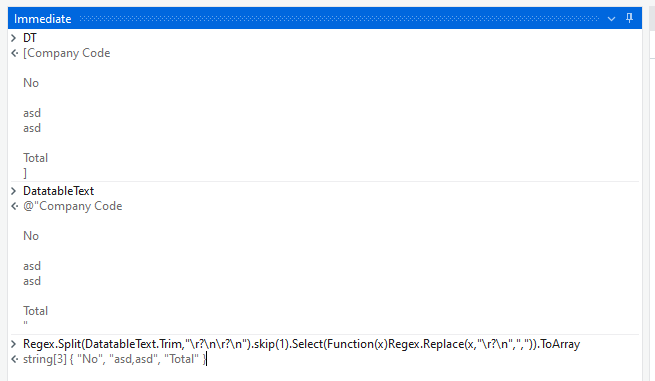# I want to Split excel rows with blank and stored in array

Hi folks,
I Have excel file and i want to split the rows with blank rows and stored in array of string.

let me know how we can split the rows in excel.

for your reference i am attaching scrrenshot.

We could maybe use the below Expression to get the column values as Array or a List :

``````companyCodeList = DT.AsEnumerable.Where(Function(x)Not String.IsNullOrWhiteSpace(x("Company Code").ToString)).Select(Function(x)x("Company Code").ToString).ToArray
``````

Here, `DT` is the Input Datatable received as the Output of `Read Range Workbook` activity and `companyCodeList` is of type Array of String.

@Akash_Javalekar1

dt1.AsEnumerable.Select(Function(r) r(“Company Code”).ToString).Where(Function(a) string.IsNullOrEmpty(a.ToString)).ToArray

Try this

`DT.AsEnumerable().Where(Function(row) Not String.IsNullOrWhiteSpace(row.Field(Of String)("columnName"))).Select(Function(row) row.Field(Of String)("columnName")).ToArray()`

Hi Thanks But i want to store
ABC1,ABC2,ABC3 stored in array(0) and ABC stored in array(1) like these one i want to stored in array of string.

In that case, could you maybe try with the below methods :

1. We could convert the Datatable value to a Text, as it contains/needed is only a Single column.

2. We could then use the text value of Datatable to perform Regex operations and get the values in required format.

From Debug :Expression to retrieve the values in segments like shown :

``````Regex.Split(DatatableText.Trim,"\r?\n\r?\n").skip(1).Select(Function(x)Regex.Replace(x,"\r?\n",",")).ToArray
``````

Here, `DatatableText` is the Output of `Output Datatable` activity and `DT` is the Input Data from Excel sheet.

Hi brother its showing regex is not declared?

Hi, you have to import namespace system.Text.RegularExpressions